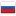Trigonometry proof [CLOSED FAST :D]

Revision en5, by Azret, 2016-06-06 13:19:04

Hello.

I am trying to prove that sin (α - β) = sin α * cos β - cos α * sin β but getting something wrong.OPα = (cos α, sin α)
OPβ = (cos β, sin β)
|OPα × OPβ| = |OPα| * |OPβ| * sin (γ)
|OPα| = |OPβ| = 1
γ = α - β
Hence, |OPα × OPβ| = sin (α - β)
Since, |A × B| = Ax * By - Ay * Bx
|OPα × OPβ| = cos α * sin β - sin α * cos β
Hence, sin (α - β) = cos α * sin β - sin α * cos β

But all formulas in internet say that sin (α - β) = sin α * cos β - cos α * sin β , i.e reverse of what I wrote above. Can someone find my mistake?

P.S Below is the correct proof written in white. You can find my mistake yourself first. ;)
As I understood I made mistake in computing angle. Since angles are + in counter-clockwise direction then
|OPα × OPβ| = ... * sin(360 - γ)
|OPα × OPβ| = |OPα| * |OPβ| * ( - sin (γ))
Hence, |OPα × OPβ| =  - sin (α - β)
And  - sin (α - β) = cos α * sin β - sin α * cos β
Leads to sin (α - β) = sin α * cos β - cos α * sin β#### History

RevisionsRev. Lang. By When Δ Comment
ru5Azret 2016-06-06 15:55:48 131
en5Azret 2016-06-06 13:19:04 131 Tiny change: 'e?\n\nP.S Here is the co' -> 'e?\n\nP.S Below is the co'
ru4Azret 2016-06-06 13:02:42 558
en4Azret 2016-06-06 13:01:41 558
en3Azret 2016-06-06 12:33:22 17 Tiny change: 'llo.\n\nI was proving that $\s' -> 'llo.\n\nI am trying to prove that$ \s'
ru3Azret 2016-06-06 12:33:04 17 Мелкая правка: 'llo.\n\nI was proving that $\s' -> 'llo.\n\nI am trying to prove that$ \s'
ru2Azret 2016-06-06 12:32:37 4
en2Azret 2016-06-06 12:32:13 4
en1Azret 2016-06-06 12:31:51 1008 Initial revision for English translation
ru1Azret 2016-06-06 12:31:09 1008 Первая редакция (опубликовано)